# A Geometric Series Problem with Shifting Indicies

Previous: Finding the Sum of an Infinite Series

Next: Koch Snowflake Example

## Question

Find the sum of the series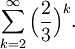## Complete Solution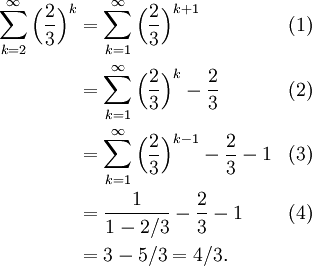## Discussion of Each Step

### Step (1)

Our overall goal is to convert the given series into the form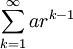so that we can apply our formula for the sum of a convergent geometric series. We can begin by shifting the index of summation from 2 to 1This will allow us to use our formula for the sum of a geometric series, which uses a summation index starting at 1. But we still cannot use our formula, because we need to have our exponent equal to k-1, and it is currently k+1.

### Step (2)

Next, we can shift the exponent down by one, to allow us to reduce the exponent by one:This requires that we subtract the term corresponding to the k=1 term.

### Step (3)

We repeat the process described in the previous step to obtain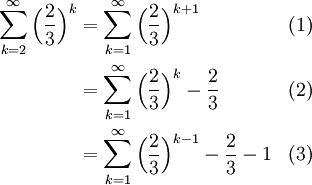### Step (4)

Now that our infinite series is in our desired form (k starts at 1 and the exponent is k-1), we apply our summation formula with a = 1 and r = 2/3,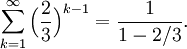The rest of the problem is algebraic manipulation.

## Possible Mistakes and Challenges

### Getting started

Students should immediately recognize that the given infinite series is geometric with common ratio 2/3, and that it is not in the form to apply our summation formula,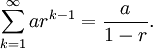To convert our series into this form, we can start by changing either the exponent or the index of summation. In the above solution, we started by changing the index of summation (students may want to, as an additional exercise, try to solve this problem by first changing the exponent).

### Shifting The Exponent

Our method for shifting the exponent in Steps (3) and (4) may cause some confusion for students. Step (3) is written out in more detail: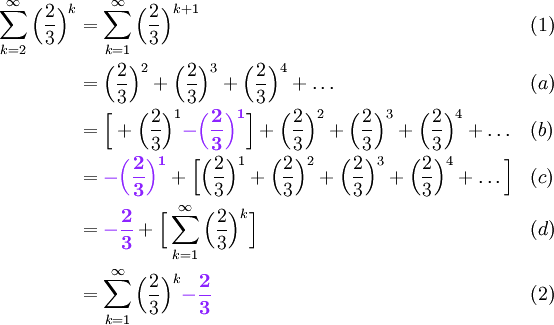In Step (b) above, we added and subtracted the same term, that term being the common ratio raised to the exponent 1. As mentioned above, the same process for shifting the exponent is used for Step (4).

Shifting of exponents was explained in the previous lesson.

Previous: Finding the Sum of an Infinite Series

Next: Koch Snowflake Example

### 1 Response to A Geometric Series Problem with Shifting Indicies

1.caper says:

Wouldn’t it have been simpler to use:
series: 4/9 + (4/9)(2/3) + …
a = 4/9
r = 2/3
a / (1 – r) = (4/9) / (1 – (2/3)) = (4/9) / (1/3) = 12/9 = 4/3 ??

Seems like steps 2 & 3 introduce a lot of potential for errors, no?
The above bypasses steps 2 & 3 altogether:
Write the 1st term: 4/9